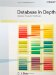# Propositions

 Recall from Chapter 4 that a proposition is a statement that's unequivocally either true or false. Given some specified set of propositions, we can combine propositions from that set in a variety of ways to form further propositions, using the connectives NOT, AND, OR, IF...THEN...(or IMPLIES), and IF AND ONLY IF (or BI-IMPLIES, or IS EQUIVALENT TO, or simply IFF). For example, here are some of the many propositions that can be formed from the set of propositions (a) Jupiter is a star, (b) Mars has two moons, and (c) Venus is between Earth and Mercury: (Jupiter is a star) OR (Mars has two moons) (Jupiter is a star) AND (Jupiter is a star) (Venus is between Earth and Mercury) AND NOT (Jupiter is a star) IF (Jupiter is a star) THEN (Mars has two moons) Clearly, the connectives can be regarded as logical operators: they take propositions as their input and return another proposition as their output. (I've used parentheses in the examples to make the scope of the connectives clear. In practice, we adopt certain precedence rules that allow us to omit many of the parentheses that might otherwise be needed. Of course, it's never wrong to include them, even when they're logically unnecessary.) A proposition that involves no connectives at all is called a simple proposition; a proposition that isn't simple is called compound. The truth value of a compound proposition can be determined from the truth values of the constituent simple propositions in accordance with the familiar truth tables:For space reasons I've abbreviated TRUE and FALSE to just T and F, respectively. By way of example, consider the following compound proposition: IF (Mars has two moons) THEN (Venus is between Earth and Mercury) This proposition is of the form IF p THEN q (or p IMPLIES q). Both constituent simple propositions evaluate to TRUE; the truth table for IMPLIES therefore shows us that the overall proposition evaluates to TRUE also. However, a point arises here that sometimes causes confusion. In the real world, the fact that Venus is between Earth and Mercury obviously has nothing to do with whether Mars has two moons. In logic, however, we simply define IF p THEN q to be true if q is true, regardless of whether p is true; in fact, if p is false, we define IF p THEN q to be true, regardless of the truth value of q. Thus, for example, IF (Jupiter is a star) THEN (Earth has two moons) is true! You can interpret this state of affairs as saying, in effect, that if you'll believe a falsehood (p), then you'll believe anything (q). NOTEIf this discussion leaves you with a slightly unpleasant taste in your mouth, you're not alone. But logicians much better than I have struggled with the problem of trying to justify the fact that IF p THEN q is true if p is false and q is true. The issue isn't worth fighting over for the purposes of this appendix. It follows from the foregoing that the proposition p IMPLIES q (or IF p THEN q) is logically equivalent to the proposition (NOT p) or q it evaluates to FALSE if and only if p evaluates to TRUE and q to FALSE. This equivalence illustrates the point that the connectives NOT, AND, OR, IMPLIES, and IFF aren't all primitive. In fact, all possible logical connectives involving either one or two propositions can be expressed in terms of suitable combinations of NOT and either AND or OR. (Exercise: Check this claim.) Perhaps even more remarkably, all such connectives can in fact be expressed in terms of just one primitive. Can you find it?Database in Depth: Relational Theory for Practitioners
ISBN: 0596100124
EAN: 2147483647
Year: 2006
Pages: 127
Authors: C.J. Date

Similar book on Amazon